Home

# Injectivity of the Connecting Maps in AH Inductive Limit Systems

## Abstract

Let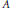$A$ be the inductive limit of a system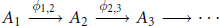$${{A}_{1\,}}\,\xrightarrow{{{\phi }_{1,\,2}}}\,{{A}_{2}}\,\xrightarrow{{{\phi }_{2,\,3}}}\,{{A}_{3}}\,\to \cdots$$

with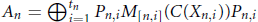${{A}_{n}}\,=\,\oplus _{i=1}^{{{t}_{n}}}\,{{P}_{n,\,i}}{{M}_{\left[ n,\,i \right]}}(C({{X}_{n,\,i}})){{P}_{n,\,i}}$ , where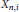${{X}_{n,\,i}}$ is a finite simplicial complex, and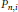${{P}_{n,\,i}}$ is a projection in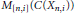${{M}_{[n,i]}}\,\left( C\left( {{X}_{n,i}} \right) \right)$ . In this paper, we will prove that$A$ can be written as another inductive limit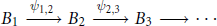$${{B}_{1}}\,\xrightarrow{{{\psi }_{1,\,2}}}\,{{B}_{2}}\,\xrightarrow{{{\psi }_{2,\,3}}}\,{{B}_{3}}\,\to \cdots$$

with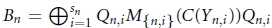${{B}_{n}}\,=\,\oplus _{i=1}^{{{s}_{n}}}\,{{Q}_{n,i}}{{M}_{\left\{ n,\,i \right\}}}(C({{Y}_{n,\,i}})){{Q}_{n,\,i}}$ , where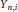${{Y}_{n,\,i}}$ is a finite simplicial complex, and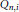${{Q}_{n,\,i}}$ is a projection in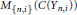${{M}_{\left\{ n,\,i \right\}}}(C({{Y}_{n,\,i}}))$ , with the extra condition that all the maps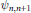${{\psi }_{n,n+1}}$ are injective. (The result is trivial if one allows the spaces${{Y}_{n,\,i}}$ to be arbitrary compact metrizable spaces.) This result is important for the classification of simple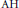$\text{AH}$ algebras. The special case that the spaces${{X}_{n,\,i}}$ are graphs is due to the third author.

• # Send article to Kindle

Note you can select to send to either the @free.kindle.com or @kindle.com variations. ‘@free.kindle.com’ emails are free but can only be sent to your device when it is connected to wi-fi. ‘@kindle.com’ emails can be delivered even when you are not connected to wi-fi, but note that service fees apply.

Find out more about the Kindle Personal Document Service.

Injectivity of the Connecting Maps in AH Inductive Limit Systems
Available formats
×

# Send article to Dropbox

To send this article to your Dropbox account, please select one or more formats and confirm that you agree to abide by our usage policies. If this is the first time you use this feature, you will be asked to authorise Cambridge Core to connect with your <service> account. Find out more about sending content to Dropbox.

Injectivity of the Connecting Maps in AH Inductive Limit Systems
Available formats
×

# Send article to Google Drive

To send this article to your Google Drive account, please select one or more formats and confirm that you agree to abide by our usage policies. If this is the first time you use this feature, you will be asked to authorise Cambridge Core to connect with your <service> account. Find out more about sending content to Google Drive.

Injectivity of the Connecting Maps in AH Inductive Limit Systems
Available formats
×

## References

Hide All
MathJax
MathJax is a JavaScript display engine for mathematics. For more information see http://www.mathjax.org.

# Injectivity of the Connecting Maps in AH Inductive Limit Systems

## Metrics

### Full text viewsFull text views reflects the number of PDF downloads, PDFs sent to Google Drive, Dropbox and Kindle and HTML full text views.

Total number of HTML views: 0
Total number of PDF views: 0 *Loading metrics...

### Abstract viewsAbstract views reflect the number of visits to the article landing page.

Total abstract views: 0 *Loading metrics...

* Views captured on Cambridge Core between <date>. This data will be updated every 24 hours.

Usage data cannot currently be displayed